# Implied Volatility and Vertical Spread Profitability###### byAleksey Tatsitov

In this article we’ll talk about how changes in implied volatility translates the profits and losses for the vertical spread strategies. This is a very important topic because it is often taught incorrectly.

## Contents

How do changes in implied volatility play a role in the performance of vertical spreads? The answer lies with extrinsic value. So, implied volatility actually measures how much extrinsic value is being priced into a stock’s options.

The figures below (Figure 1, 2) represent different levels of implied volatility and its changes over the time.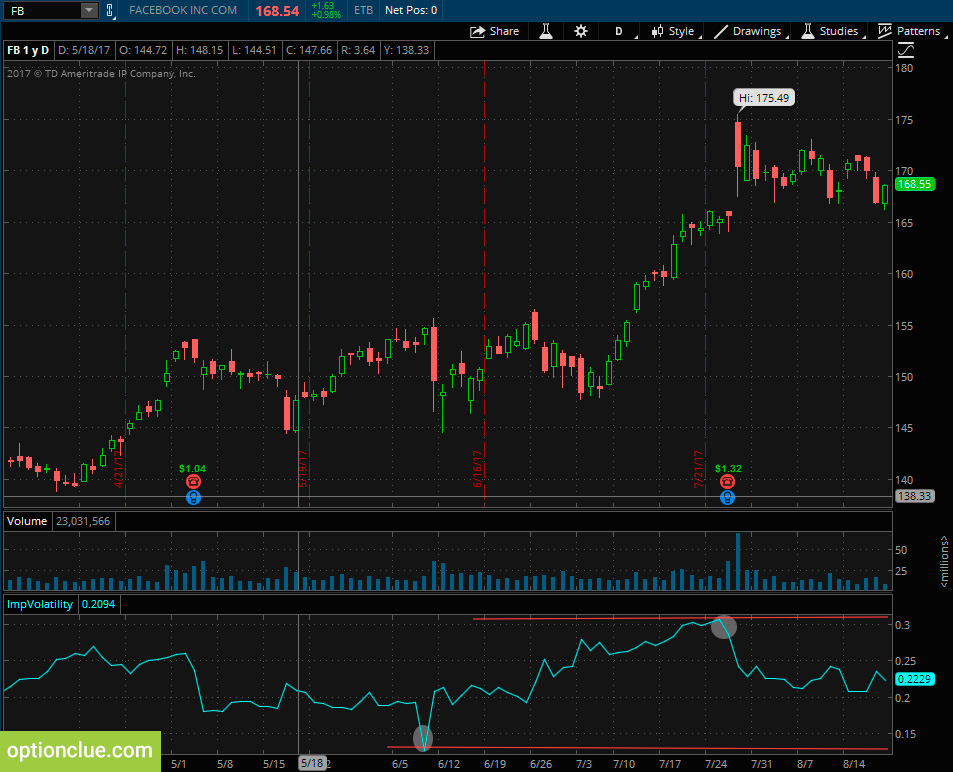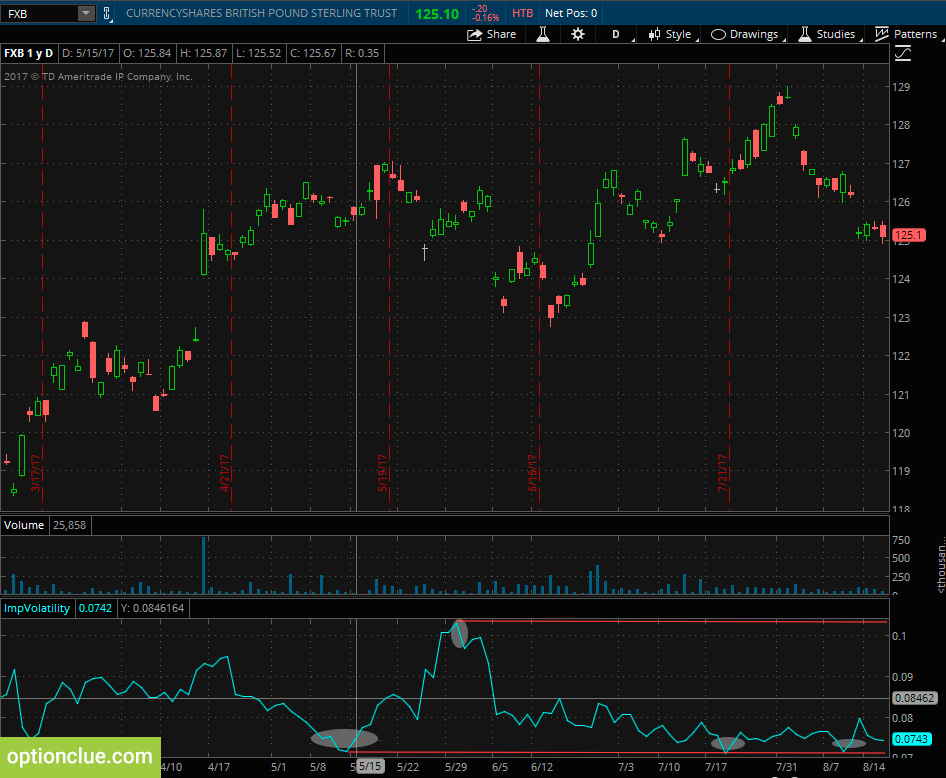Figure 2. FXB chart. Implied volatility indicator (thinkorswim trading platform)

Stocks that are traded with higher levels of implied volatility have options with more extrinsic value than stocks that are trading with lower levels of implied volatility.

Lower or higher implied volatility comes from the extrinsic value in the stocks options, which comes from the amount of uncertainty the market is pricing into that stocks future price movements.

How does this relate to trading vertical spreads? As you know, a vertical spread can only reach maximum profit if the extrinsic value in the spread reaches zero. That means that you want implied volatility to decrease as the stock is moving in favor of your spread.

Stocks that are traded with higher levels of implied volatility have options with more extrinsic value in stocks with lower levels of implied volatility and have options with less extrinsic value.

So if that high implied volatility stock transitions into a lower implied volatility stock then that just means that the options on that stock are trading with less extrinsic value than they did previously.

When you’re trading vertical spreads you want a favorable directional movement combined with a decrease in implied volatility.

This is exactly the opposite of what many other people will tell you in terms of trading vertical spreads.

And that is just not true. In fact if you’re trading a vertical spread and the stock moves in favor of your spread while implied volatility is increasing you’ll actually have less profits than you would have with a decrease in implied volatility or if implied volatility had just stayed flat.

With that said there is a time in which an increase in implied volatility will actually be a good thing for your position and that’s only if the stock price is moving against your spread.

For example if you buy a call spread and the stock price decreases then an increase in implied volatility will actually dampen the losses on that spread. But if implied volatility increases and the stock is moving in favor of your spread you’ll actually be worse off.

We’re going to walk through some examples in order to look at Profit/Loss estimations based on changes in implied volatility, and we’re going to see how it influences the performance of these vertical spreads.

## Changes in Implied Volatility

For this example, we’re going to look at Apple options. Apple is traded at \$161.60. For this demonstration, we’re going to look at October options with 65 days until expiration (Figure 3).

The first trade I want to look at is the 160-170 bull call spread. We’re buying the 160 call and then we’re selling the call in the October expiration cycle. So we entered this trade for \$4.33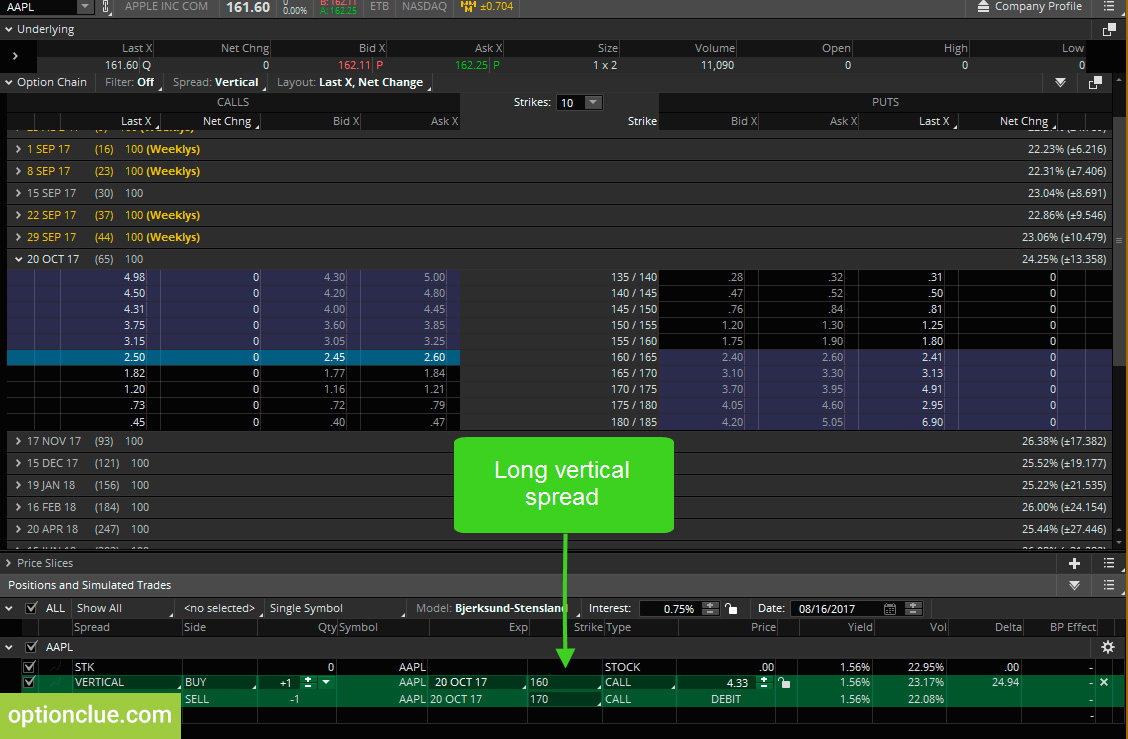Figure 3. Apple option chain (thinkorswim trading platform)

In the risk profile we can see here this first dotted line is the approximate location of the long calls strike price of \$160 and this second line is the location of the short call strikes so that in this case is \$170. Now we can see two curves here.
This flatter blue line actually represents the PL estimation of this call spread if Apple’s implied volatility were to increase by 22% (Figure 4).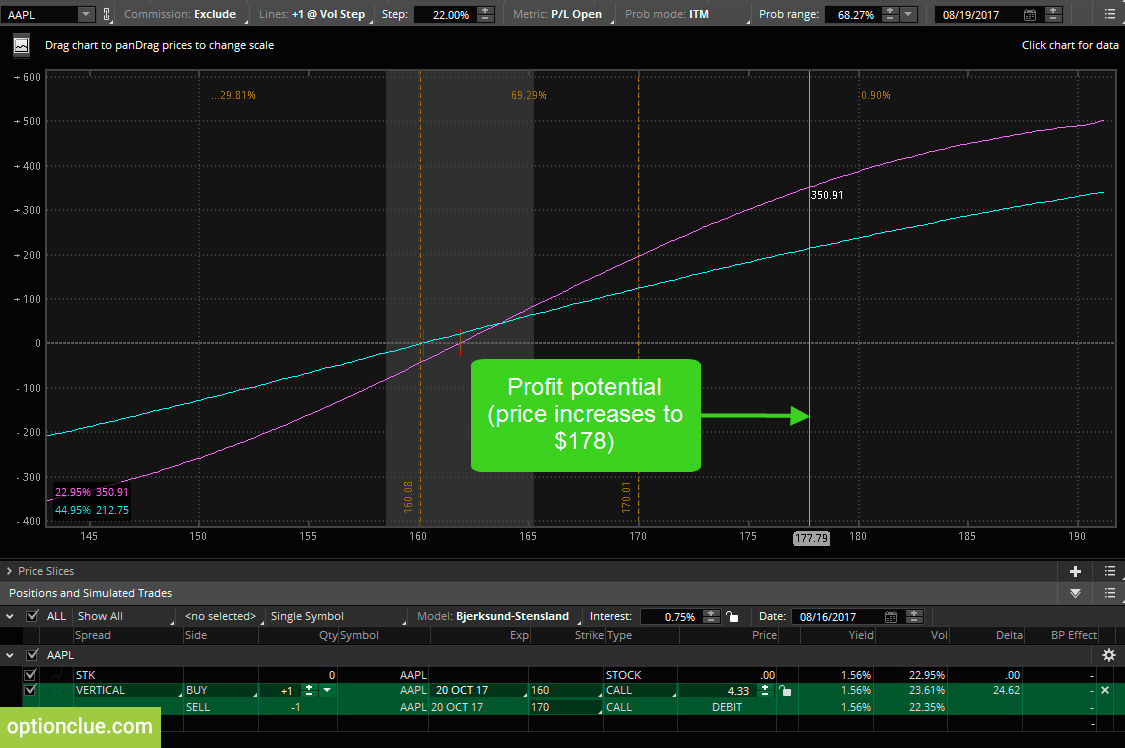Figure 4. PL estimation when the price increases (thinkorswim trading platform)

We can see that as of now Apple is trading with an implied volatility of around 22.9 % but this blue flatter line is estimating the profitability of this spread if Apple is implied volatility were to 44.9%. These two numbers in the lower left hand corner is what we want to pay attention to.

We’ve queued up the 160-170 long call spread or Bull call spread and as we can see here let’s say the stock price goes to \$177 tomorrow so with the stock price at \$177 based on the numbers in the lower left-hand corner we can see that if implied volatility does not change we expect to have a profit of around \$350.

However, if implied volatility increases by 22% to 44% we only expect to have a profit of \$212. As I said earlier a lot of people will tell you that when you buy a call spread or buy a put spread you want implied volatility to increase because that’s going to help your position.

But as we can see in the example if the stock price goes above our short call strike price of 170 and implied volatility doubles we’re going to be much worse off than we would have been if implied volatility had remained the same.

Now on the other hand what if the stock price falls to 152 dollars.

If the stock price falls to 152 dollars we expect to be down about \$228 with implied volatility unchanged at 22.9% but if implied volatility skyrockets up to 44.9% we only expect to be down about \$108 (Figure 5).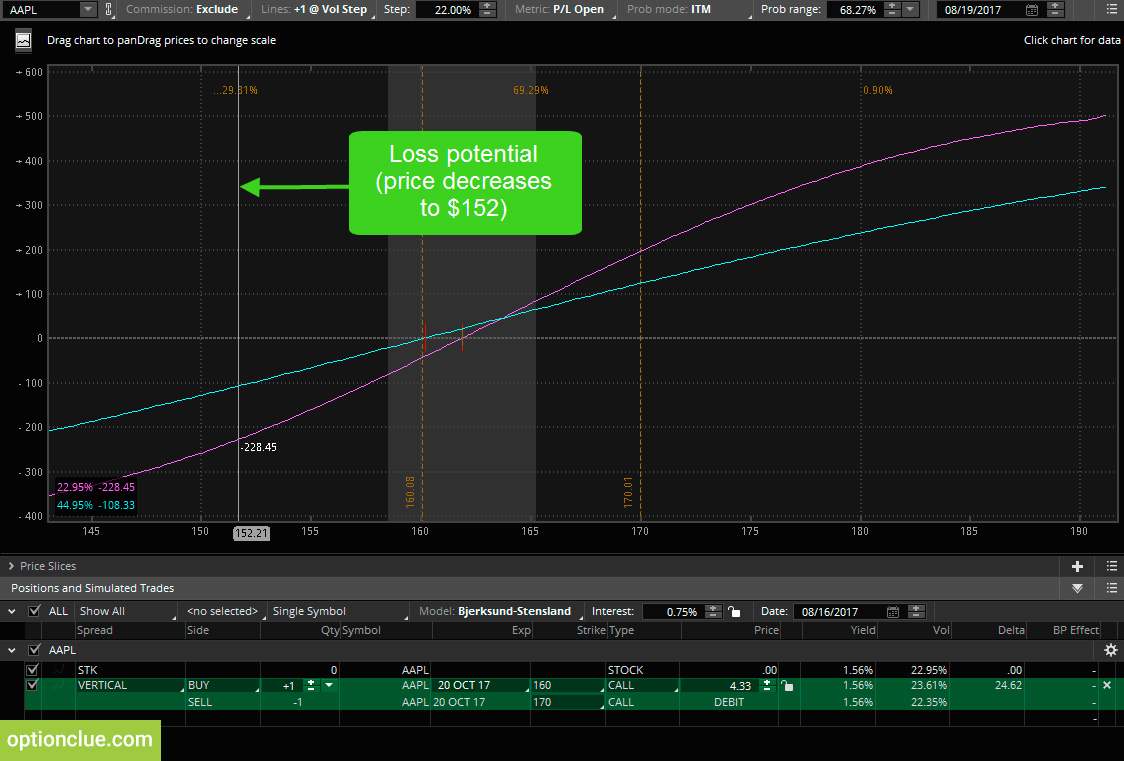Figure 5. PL estimation when the price decreases (thinkorswim trading platform)

So when you buy a call spread or buy a put spread and the stock price moves against you an increase in implied volatility should help lessen the losses on that trade. However, if the stock price moves in favor of your spread you want implied volatility to decrease because that is an indication that the extrinsic value of those options is decreasing.

## Zero Implied Volatility and Vertical Spread PL Estimation

Now I’m actually going to drop down this implied volatility number and put in negative 22%. I did that because the current implied volatility in Apple is 22.95% and I want to look at the estimation of profits and losses for the spread if implied volatility were to go to 0% (Figure 6).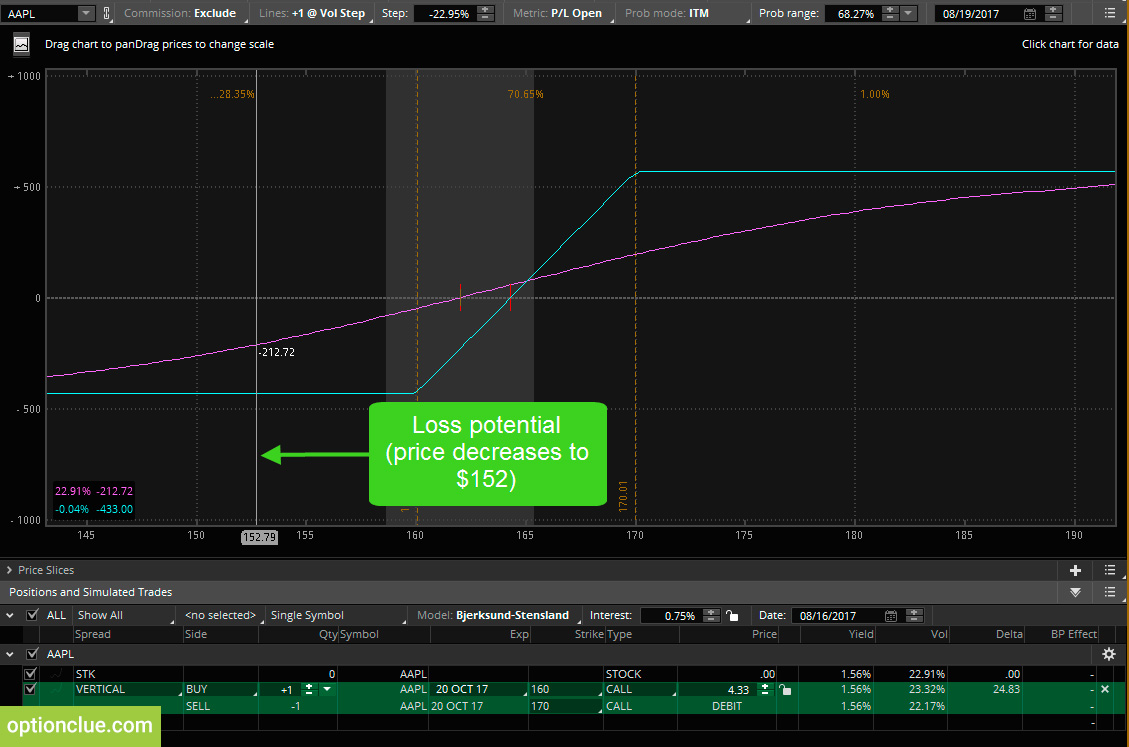Figure 6. Max loss. Implied volatility is 0% (thinkorswim trading platform)

As we can see if implied volatility goes to 0% if the stock price is below \$160 which is the long call strike of this particular call spread then we expect to lose \$433. That makes sense because our maximum loss potential is \$433.

On the other hand, if the stock price goes to any price above \$170, which is the short called strike price, then we expect to have a profit of around \$567 (Figure 7).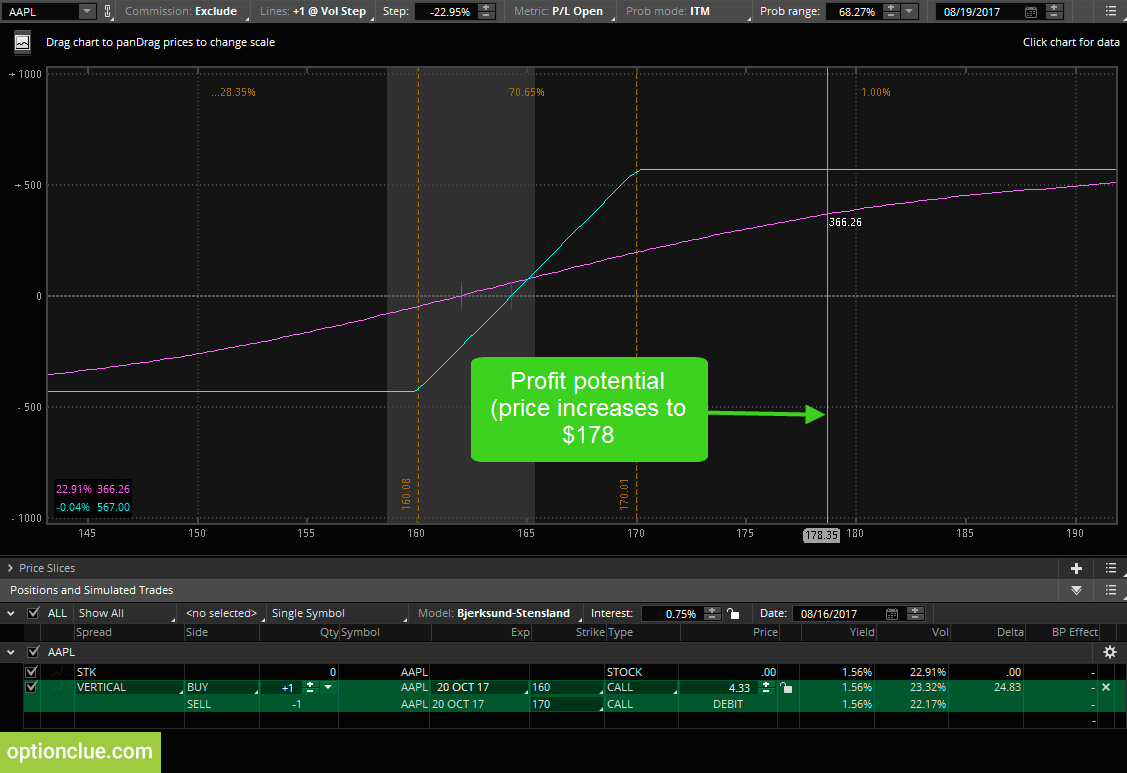Figure 7. Max profit. Implied volatility is 0% (thinkorswim trading platform)

That’s pretty much the maximum profit potential of this trade. So clearly if the stock price moves in favor of your spread which in this case would be an increase to a price equal to or above \$170 a decrease in implied volatility will greatly help the profitability of this spread.

That’s because a decrease in implied volatility just means that the extrinsic value of the options is being traded away essentially.

So, if a stock is trading with 0% implied volatility that means that the options have no extrinsic value and if the options have no extrinsic value that means that the market is pricing in absolute certainty in regards to where the stock price will close at the time that these options expire.

When the stock price moves in favor of your vertical spread you want to get a decrease in implied volatility because that means extrinsic value is decreasing, however, when the stock price moves against your vertical spread, you want an increase in implied volatility because that means the options will have more extrinsic value.

## Summary

These two demonstrations really helped you understand how implied volatility plays a massive part in the profitability of vertical spreads.

Since vertical spreads require a decrease in extrinsic value to reach the maximum profit potential you want implied volatility to decrease as the stock price is moving in your favor and as we just discussed if the stock price moves against your vertical spread an increase in implied volatility may result in less severe losses and that’s because an increase in implied volatility is an indication that extrinsic value is increasing.

Share